# triangle free

## Bounding the chromatic number of triangle-free graphs with fixed maximum degree ★★

Author(s): Kostochka; Reed

Conjecture   A triangle-free graph with maximum degreehas chromatic number at most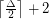.

Keywords: chromatic number; girth; maximum degree; triangle free

## Non-edges vs. feedback edge sets in digraphs ★★★

Author(s): Chudnovsky; Seymour; Sullivan

For any simple digraph, we let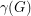be the number of unordered pairs of nonadjacent vertices (i.e. the number of non-edges), and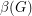be the size of the smallest feedback edge set.

Conjecture  Ifis a simple digraph without directed cycles of length, then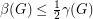.

Keywords: acyclic; digraph; feedback edge set; triangle free

## Circular coloring triangle-free subcubic planar graphs ★★

Author(s): Ghebleh; Zhu

Problem   Does every triangle-free planar graph of maximum degree three have circular chromatic number at most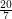?

Keywords: circular coloring; planar graph; triangle free

## Unions of triangle free graphs ★★★

Author(s): Erdos; Hajnal

Problem   Does there exist a graph with no subgraph isomorphic to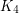which cannot be expressed as a union oftriangle free graphs?

## Triangle free strongly regular graphs ★★★

Author(s):

Problem   Is there an eighth triangle free strongly regular graph?

Keywords: strongly regular; triangle free